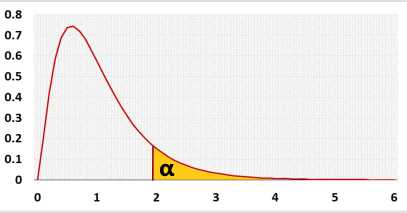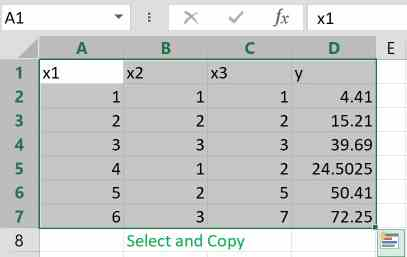# Linear Regression Calculator

## Multiple Variables

The calculator uses an unlimited number of variables, calculates the Linear equation, R, p-value, outliers and the adjusted Fisher-Pearson coefficient of skewness.
After checking the residuals' normality, multicollinearity, homoscedasticity and priori power, the program interprets the results.
Then, it draws a histogram, a residuals QQ-plot, a correlation matrix, a residuals x-plot and a distribution chart.
You may transform the variables, exclude any predictor or run backward stepwise selection automatically based on the predictor's p-value.

## Hypotheses

 H0: Y=b0 H1: Y=b0+b1X1+...+bpXp

## Test statistic## F distribution## Test calculation

### Statistic Data

 Outliers: IncludedExcluded Effect size f2:Expected R2: The power is expected to identify the effect. If one exists, H0 will be rejected. effect size f2 - Cohen's effect size f2, the ratio between the explained variance and the unexplained variance. f2=R2/(1-R2)examples:0.02 - small effect, 0.15 - medium , 0.35 - largeR2 - the expected R Squared, the ratio of the explain variance Iterations ManuallyAutomatically Automatic will remove the most insignificant variable and run another iteration until all Xi variables are significant or only one variable left α: Significant level (0-1), maximum chance allowed rejecting H0 while H0 is correct (Type1 Error) Digits: 12345676910 When choosing 2 digits, 0.00001234 will be rounded to 0.000012

Enter raw data directly
Enter raw data from excel

## Enter sample data directly

Header: You may change the groups' names to their real names.
Data: When entering data, press Enter or comma ',' after each value.
* All variables will be included in the automatic iterations mode.
** Normality colors based on α=0.05

 *Include ✔✘ ✔✘ Transform LogLnSqrt^2 LogLnSqrt^2 LogLnSqrt^2 Groups X1 X2 Y Data pval: avg: n: S: Skewness: Normality:** Outliers:
 Groups Pred Y Residual Data pval: avg: n: S: Skewness Normality Outliers

## Enter sample data from excel

You may copy data from Excel, Google sheets or any tool that separate data with Tab and Line Feed.
Copy the data, one block of consecutive columns includes the header, and paste below. Y must be the right column. . click to see example:Correlation matrix
ANOVA table
anova
tresults2

.
validation message